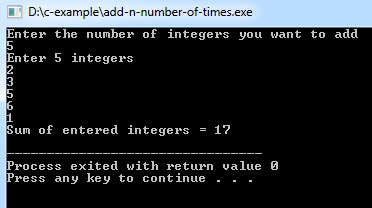# C Program to Add n Number of Times

This C program adds n number of times which will be entered by the user.

Program:
``````#include <stdio.h>

int main()
{
int n, sum = 0, c, value;

printf("Enter the number of integers you want to add\n");
scanf("%d", &n);

printf("Enter %d integers\n",n);

for (c = 1; c <= n; c++)
{
scanf("%d",&value);
sum = sum + value;
}

printf("Sum of entered integers = %d\n",sum);

return 0;
}``````
Program Output:Explanation:

Here is a program that shows how to add n number given by the user. So first of all, you have to include the stdio header file using the "include" preceding #, which tells that the header file needs to be process before compilation, hence named preprocessor directive.

Then you have to define the main() function, and it has been declared nothing, so by default, it returns an integer. Now, you have to first take four integer type variable names as 'n', 'sum', 'c', and 'value' and initialize sum as 0. Now you have to display a message using printf() function - "Enter the number of integers you want to add". The scanf() function will fetch a value from the user and store it in 'n'. Again another printf() which will show the message on the screen - "Enter %d integers.

Now a for loop will be required, which will count the value from '1' to 'n' using variable 'c', and using scanf() takes the values one by one. The inserted value will then be added up with the variable sum so that the program can yield the total of all the numbers.

In the end, the return 0; statement is used to return an integer type value back to main().

## Same C Program Using an Array

Program:
``````#include <stdio.h>

int main()
{
int n, sum = 0, c, array;

scanf("%d", &n);

for (c = 0; c < n; c++)
{
scanf("%d", &array[c]);
sum = sum + array[c];
}

printf("Sum of entered integers = %d\n",sum);

return 0;
}``````